2014
12-23

# 史上最全淘宝各类点击扣费价格

1.电商搜索页面，淘宝为例：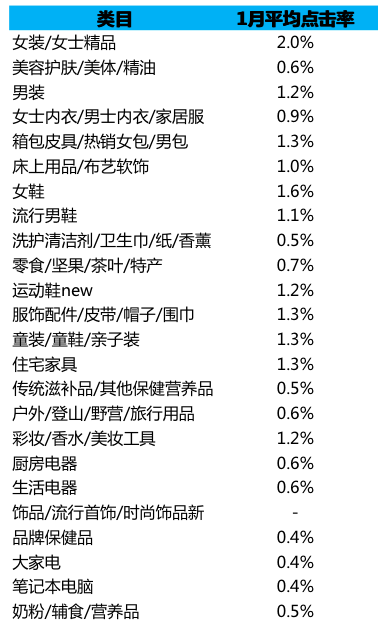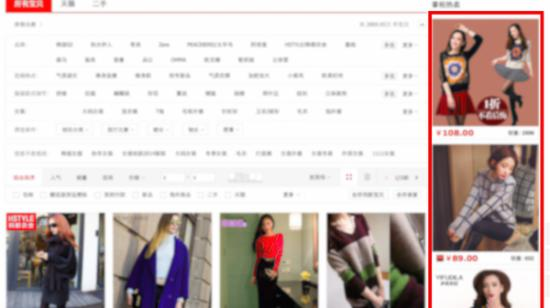CTR（点击率）在2%，即百次展现点击2次

CTR*CPC*17+CPM*0.001*3

0.02*3*17+10*0.001*3=1.05

2.电商首页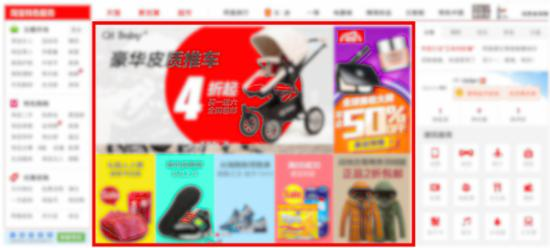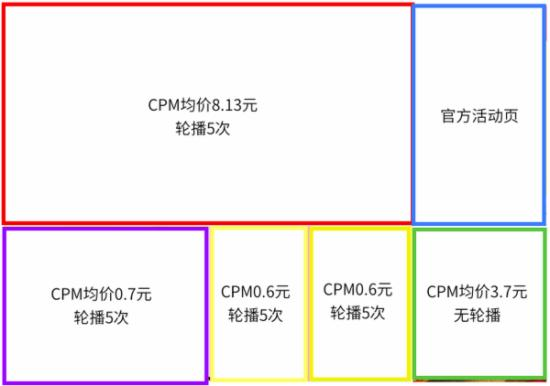((CPM1+CPM2+CPM3+CPM4)*5+CPM5)*0.001/0.8

((8.13+0.7+0.6+0.6)*5+3.7)*0.001/0.8=0.0673125

3.视频：优酷为例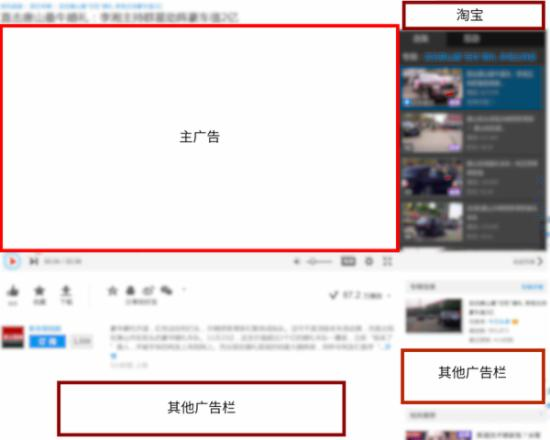CPM*4*0.001/0.8=0.15

4.百度搜索页面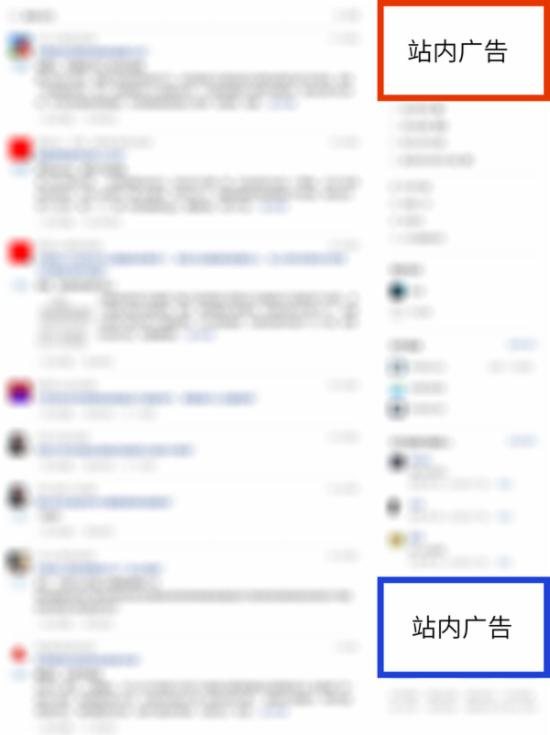CPM*4*0.001/0.8=0.15

4.百度搜索页面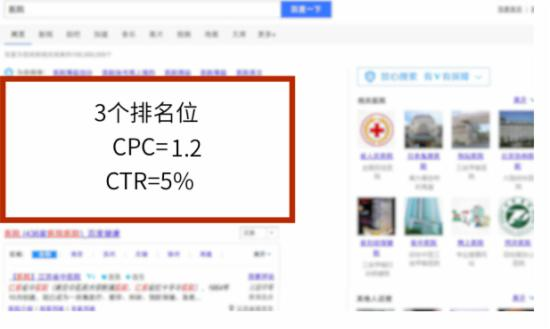CTR1*3*CPC1+CTR*CPC2*8

0.05*1.2*3+0.005*0.5*8=0.2

5.QQ聊天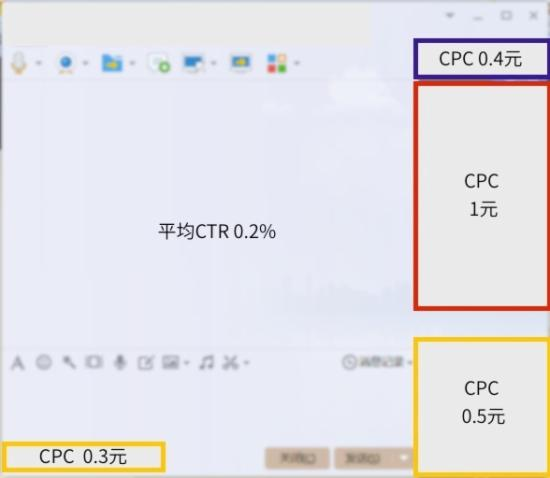(CPC1+CPC2+CPC3+CPC4)*CTR

(0.3+0.5+1+0.4)*0.002=0.0044

6.知乎7.移动端：淘宝APP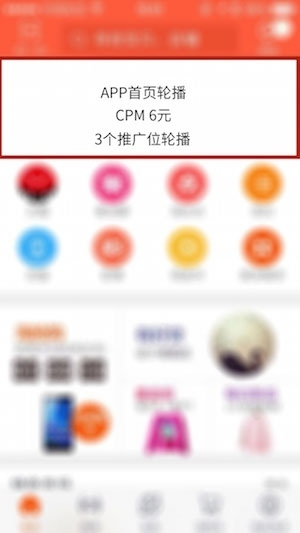CPM*0.001*3/0.8

8.微信丶手机QQ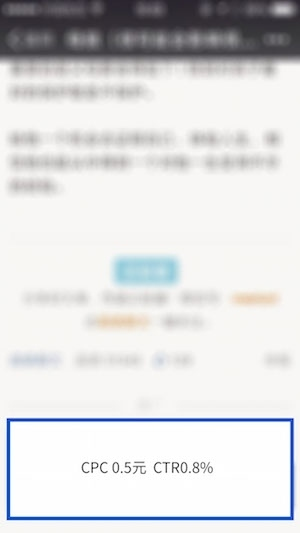CPC*CTR